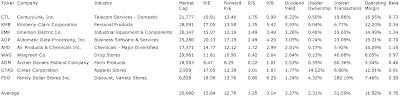## Pages

### Dividend Aristocrats With Biggest Insider Buys

Dividend Aristocrats With Highest Insider Transactions by Dividend Yield - Stock, Capital, Investment. Here is a current sheet of stocks from the Dividend Aristocrats index, a product of Standard & Poor’s, that have shown a significant rise in insider ownership by more than 5 percent over the recent six months. 9 stocks fulfilled these criteria of which 4 have a yield of more than 3 percent. The biggest hike was realized by Family Dollar Stores (NYSE:FDO) where insiders increased their stake by 284.7 percent.

Here are the 3 top dividend stocks by yield:

CenturyLink (NYSE:CTL) has a market capitalization of \$21.78 billion. The company employs 49,250 people, generates revenues of \$7,041.53 million and has a net income of \$949.13 million. The firm’s earnings before interest, taxes, depreciation and amortization (EBITDA) amounts to \$3,493.24 million. Because of these figures, the EBITDA margin is 49.61 percent (operating margin 29.25 percent and the net profit margin finally 13.48 percent).

The total debt representing 33.25 percent of the company’s assets and the total debt in relation to the equity amounts to 76.00 percent. Due to the financial situation, the return on equity amounts to 9.92 percent. Finally, earnings per share amounts to \$1.78 of which \$2.90 were paid in form of dividends to shareholders last fiscal.

Here are the price ratios of the company: The P/E ratio is 19.83, Price/Sales 3.10 and Price/Book ratio 1.12. Dividend Yield: 8.20 percent. The beta ratio is 0.73.Long-Term Stock Chart Of CenturyLink, Inc. (Click to enlarge)

Kimberly Clark (NYSE:KMB) has a market capitalization of \$28.09 billion. The company employs 57,000 people, generates revenues of \$19,746.00 million and has a net income of \$1,762.00 million. The firm’s earnings before interest, taxes, depreciation and amortization (EBITDA) amounts to \$3,611.00 million. Because of these figures, the EBITDA margin is 18.29 percent (operating margin 14.04 percent and the net profit margin finally 8.92 percent).

The total debt representing 32.78 percent of the company’s assets and the total debt in relation to the equity amounts to 110.04 percent. Due to the financial situation, the return on equity amounts to 32.55 percent. Finally, earnings per share amounts to \$4.18 of which \$2.64 were paid in form of dividends to shareholders last fiscal.

Here are the price ratios of the company: The P/E ratio is 17.04, Price/Sales 1.40 and Price/Book ratio 4.83. Dividend Yield: 3.99 percent. The beta ratio is 0.34.Long-Term Stock Chart Of Kimberly Clark Corp (Click to enlarge)

Emerson Electric (NYSE:EMR) has a market capitalization of \$36.15 billion. The company employs 133,200 people, generates revenues of \$24,222.00 million and has a net income of \$2,504.00 million. The firm’s earnings before interest, taxes, depreciation and amortization (EBITDA) amounts to \$4,721.00 million. Because of these figures, the EBITDA margin is 19.49 percent (operating margin 14.99 percent and the net profit margin finally 10.34 percent).

The total debt representing 21.80 percent of the company’s assets and the total debt in relation to the equity amounts to 50.01 percent. Due to the financial situation, the return on equity amounts to 24.31 percent. Finally, earnings per share amounts to \$3.26 of which \$1.38 were paid in form of dividends to shareholders last fiscal.

Here are the price ratios of the company: The P/E ratio is 15.09, Price/Sales 1.49 and Price/Book ratio 3.48. Dividend Yield: 3.27 percent. The beta ratio is 1.24.Long-Term Stock Chart Of Emerson Electric Co. (Click to enlarge)

Here is the full table with some fundamentals (TTM):Dividend Aristocrats With Biggest Insider Buys (Click to enlarge)

Take a closer look at the full table. The average price to earnings ratio (P/E ratio) amounts to 15.84 while the forward price to earnings ratio is 12.76. The dividend yield has a value of 3.27 percent. Price to book ratio is 3.14 and price to sales ratio 1.25. The operating margin amounts to 11.92 percent. Insiders own 2.31 percent of the company and increased their stake by 51.59 percent within the past 6 months.

Related stock ticker symbols: